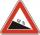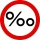# Percentages + per mil - math problems

#### Number of problems found: 6Road sign informs the gradient is 10.9%. Calculate the angle which average decreases.
• Altitude differenceWhat a climb in per mille of the hill long 4 km and the altitude difference is 6 meters?
• AMSL and skiingTomas skis from point A (3200m above sea level to place B. Hill has 20% descent. The horizontal distance between the start and finish is 2,5km. At what altitude is point B?
• CrystalThe crystal grows every month 1.2 permille of its mass. For how many months to grow a crystal from weight 177 g to 384 g?
• Climb in percentageThe height difference between points A and B is 475 m. Calculate the percentage of route climbing if the horizontal distance places A, B is 7.4 km.
• RiverCalculate how many permille river Dunaj average falls, if on section long 957 km flowing water from 1454 m AMSL to 101 m AMSL.

We apologize, but in this category are not a lot of examples.
Do you have an interesting mathematical word problem that you can't solve it? Submit a math problem, and we can try to solve it.

We will send a solution to your e-mail address. Solved examples are also published here. Please enter the e-mail correctly and check whether you don't have a full mailbox.

Please do not submit problems from current active competitions such as Mathematical Olympiad, correspondence seminars etc...